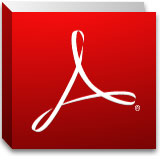You are hereCourses / Mathematics / Discrete Mathematics S1 (#451)

# Discrete Mathematics S1 (#451)

Course Description:

Discrete Mathematics 451 is a ½ semester study basic concepts of mathematics and mathematical reasoning.  This course covers the studies of problem solving and critical thinking, set theory, logic, number theory and the real number system, algebraic equations and inequalities and the graphs, functions and linear systems.

Problem Solving and Critical Thinking
a.  Inductive and Deductive Reasoning
b.  Estimation, graphs and Mathematical Models
c.  Problem Solving

Set Theory
a.  Basic Set Concepts
b.  subsets
c.  Venn diagrams and Set Operations
d.  Set Operations and Venn Diagrams with Three Sets
e.  Survey Problems

Logic
a.  Statements, Negations and Quantified Statements
b.  Compound Statements and Connectives
c.  Truth Tables for Negation, Conjunction and Disjunction
d.  Truth Tables for Conditional and Biconditional
e.  Equivalent Statements and Variations of Conditional Statements
f.  Arguments and Truth Tables

Number Theory and the Real Number System
a.  Number Theory:  Prime and Composite numbers
b.  the Integers and Order of Operations
c.  Rational and Irrational Numbers
d.  Real Number Properties
e.  Exponents and Scientific Notation
f.  Arithmetic and Geometric Sequences

Algebra:  Equations and Inequalities
a.  Algebraic Expressions and Formulas
b.  Linear Equations and Inequalities in One Variable
c.  Applications of Linear Equations

Algebra:  Graphs, Functions and Linear Systems
a.  Graphing and Functions
b.  Systems of Linear Equations in Two Variables
c.  Linear Programming
d.  Modeling Data

## Important notice regarding our English course offering:

All English courses (listed) are temporarily suspended until further notice: English I S1 (#311), English I S2 (#312), English II S1 (#321), English II S2 (#322), English III S1 (#331), English III S2 (#332), English IV S1 (#341), and English IV S2 (#342).

## Notice to Prospective StudentsStudents must have a valid e-mail address, physical mailing address and access to any free PDF file viewer, like Adobe Reader, for filling out course materials online and printing course materials at home.